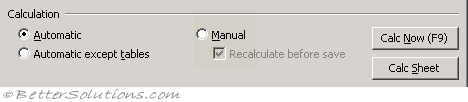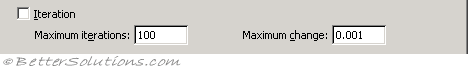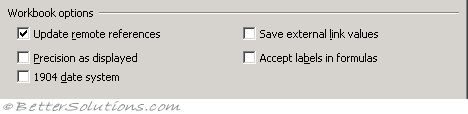# Calculation Tab

### Calculation`Application.Calculation = xlCalculation.xlCalculationManual Application.Calculation = Excel.xlManual `

The second line is only made available for backwards compatibility.

`Application.Calculation = xlCalculation.xlCalculationAutomatic Application.Calculation = Excel.xlAutomatic `

The second line is only made available for backwards compatibility.

`Application.Calculation = xlCalculation.xlCalculationSemiautomatic Application.Calculation = Excel.xlSemiautomatic `

The second line is only made available for backwards compatibility.

`Application.CalculateBeforeSave = True Application.Calculate ActiveSheet.Calculate `

If you want to calculate just a selection of cells you could use Range("A2:E10").Calculate.
For more details on VBA Calculation, please refer to the Formulas > VBA Code > Calculation

### Iteration`Application.Iteration = True Application.MaxIterations = 1000 Application.MaxChange = 0.001 `

### Workbook Options`ActiveWorkbook.UpdateRemoteReferences = True ActiveWorkbook.PrecisionAsDisplayed = True ActiveWorkbook.Date1904 = True ActiveWorkbook.SaveLinkValues = True ActiveWorkbook.AcceptLabelsInFormulas = True `

`Application.AskToUpdateLinks = True `